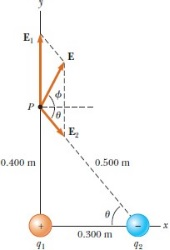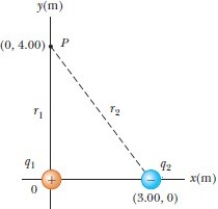# Physics Problem Set 9

1. The electron and proton of a hydrogen atom are separated (on average) by a distance of about 5.3 ×10-11 m. Find the magnitudes of the electric force and the gravitational force that each particle exerts on the other, and the ratio of the electric force Fe to the gravitational force Fg.

2. A Charge q1 = 00 μC is at the origin, and charge q2 = -5.00 μC is on the x-axis, 0.300 m from the origin (Fig. on the right).

a. Find the magnitude and direction of the electric field at point P, which has coordinates (0, 0.400) m.

b. Find the force on a charge of 00 ×10-8 C placed at P.3. An A00-μC point charge is at the origin, and a point charge q2 = - 3.00 μC is on the x-axis at (3.00, 0) m, as in the Figure. If the electric potential is taken to be zero at infinity, find the total electric potential due to these charges at point P with coordinates (0, 4.00) m.